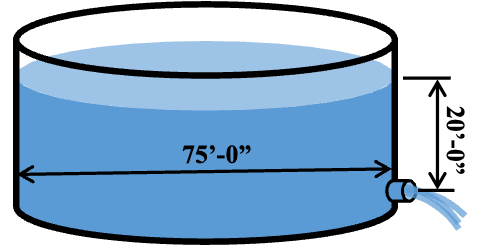# Time to Discharge Tank from Orifice

Problem solution was updated to correct formulae for flow and volume.A 75-foot diameter tank filled is being drained through a 10-inch diameter short pipe orifice.

Given that the height of water reaches 20 feet above the orifice centerline, determine the time it will take to drain the tank to the orifice level.

A) 53 min

B) 69 m in

C) 88 min

D) 96 min

Solution

Coefficient of Discharge (given):
$C_d=0.8$
Area of Orifice:
$A_0= \frac{π*d_0^2}{4}$
$A_0= \frac{π*(10 \ in)^2}{4}=0.55 \ ft^2$
Height of Fluid Above Orifice:
$h=20 \ ft$
Gravity:
$g=32.2 \ ft/s^2$

Discharge Flow from Orifice:
$Q=C_d A_0 \sqrt{2gh}$
$\sout{Q=0.85×78.5×\sqrt {2×32.2×20}}$$Q=0.85×0.55×\sqrt {2×32.2×20}$
$Q=16.6 \ cfs$
Volume of Water Above Orifice:

$V=\frac{\sout{π*d_{tank}^2}}{\sout{4*h}}$

$V=\frac{π*d_{tank}^2 *h}{4}$

$V= \frac{\sout{π*(75 \ ft)^2}}{\sout{4*20 \ ft}}=88,357.3 \ ft^3$

$V= \frac{π*(75 \ ft)^2 *20 \ ft}{4}=88,357.3 \ ft^3$

Discharge Time:

$t= \frac{V}{Q}= \frac{88,357.3 \ ft^3}{16.6 \ cfs}=5322 \ s ≈89 \ min$